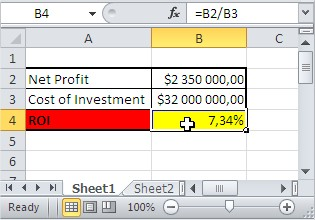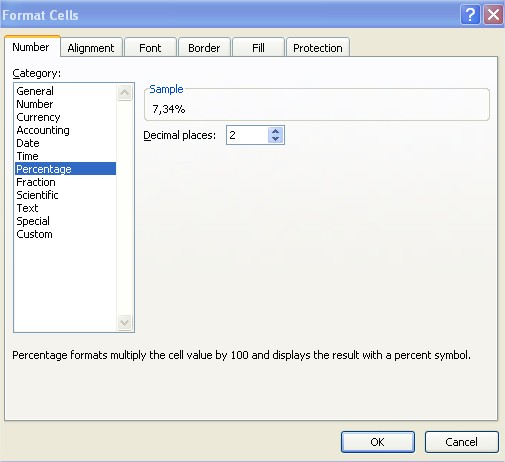#### How to calculate ROI in Excel

In this lesson, you will learn how to calculate ROI in Excel.

## What is ROI?

ROI stands for return on investment. Return on investment is one of the most basic measures in business. A ROI is a profitability indicator that is used to measure the efficiency of a company, regardless of the structure of its assets or extraordinary factors. Return on investment is also helpful for project managers because it helps them calculate the efficiency of the project.

Let’s build the return on investment calculator in Excel. To calculate ROI in Excel, first you need some data. You require a net profit and the cost of the investment.

## How to calculate?

Copy and paste this ROI formula into cell B4: =B2/B3This formula will calculate the ROI for the investment data you place in cells B2 and C2 and is based on the ROI equation formula:

ROI = Net Profit / Investment Cost

In other words, the ROI is simply the return on the amount invested, so the equation can be written alternatively like this:

ROI = (Present Value – Starting Value) / Starting Value

Remember to format the ROI as a percentage. Click on the B4 cell > CTRL + 1 keyboard shortcut > Percentage with 2 decimal places.The result is greater than 0, so this is the profit from an investment, which is 7.34%.

When the result is below zero, it is a loss.

If desired, you can add conditional formatting to highlight positive and negative ROI values. To do this, select the cell, go to the Home tab, click on the Conditional Formatting group, and select Icon Sets. From the options available, you can choose a set of symbols to display positive and negative ROI values.

Excel’s “Goal Seek” and “Solver” tools allow you to perform sensitivity analysis. You can set up scenarios by varying specific input values (e.g., discount rate or revenue growth rate) to observe their impact on ROI. This helps in understanding the sensitivity of your ROI to different variables.

## Things to remember

Remember that the return on investment result does not take into account a few important aspects that you should be aware of.

Typically, the return on investment is calculated as the market value of the investment divided by its initial value. Market value does not mean that it is the amount of cash that you are currently getting. In fact, you may find that the cash you are able to get is lower.

You should also consider the time it takes to cash in your investment, which can be long.

Return on investment is the ratio of the present value to the initial value without accounting for inflation, taxes, costs of obtaining capital or risk. The low value of the return on investment may not compensate for the risk premium or may be lower than inflation.

It is recommended to calculate the annual return on investment and compound annual growth rate as well, which can give you more information about the true success of your investment.